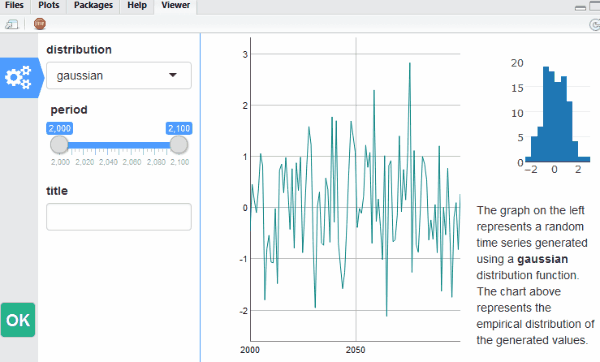# Getting started with the manipulateWidget package

#### 2020-02-24

The `manipulateWidget` package is largely inspired by the `manipulate` package from Rstudio. It provides the function ``manipulateWidget` that can be used to create in a very easy and quick way a graphical interface that lets the user modify the data or the parameters of an interactive chart. Technically, the function generates a Shiny gadget, but the user does not even have to know what is Shiny.

The package also provides the `combineWidgets` function to easily combine multiple interactive charts in a single view. Of course both functions can be used together: here is an example that uses packages `dygraphs` and `plot_ly` (code at the end of the document).An example of what one can do with manipulateWidgets

## Getting started

The main function of the package is `manipulateWidget`. It takes as argument an expression that generates an interactive chart (and more precisely an htmlwidget object. See http://www.htmlwidgets.org/ if you have never heard about it) and a set of input controls created with functions mwSlider, mwCheckbox… which are used to dynamically change values within the expression. Each time the user modifies the value of a control, the expression is evaluated again and the chart is updated. Consider the following code:

``````manipulateWidget(
myPlotFun(country),
country = mwSelect(c("BE", "DE", "ES", "FR"))
)``````

It generates a graphical interface with a select input on its left with options “BE”, “DE”, “ES”, “FR”. The value of this input is mapped to the variable `country` in the expression. By default, at the beginning the value of `country` will be equal to the first choice of the input. So the function will first execute `myPlotFun("BE")` and the result will be displayed in the main panel of the interface. If the user changes the value to “FR”, then the expression `myPlotFun("FR")` is evaluated and the new result is displayed.

The interface also contains a button “Done”. When the user clicks on it, the last chart is returned. It can be stored in a variable, be modified by the user, saved as a html file with saveWidget from package htmlwidgets or converted to a static image file with package `webshot`.

Of course, one can create as many controls as needed. The interface of the animated example in the introduction was generated with the following code:

``````manipulateWidget(
myPlotFun(distribution, range, title),
distribution = mwSelect(choices = c("gaussian", "uniform")),
range = mwSlider(2000, 2100, value = c(2000, 2100), label = "period"),
title = mwText()
)``````

To see all available controls that can be added to the UI, take a look at the list of the functions of the package:

``help(package = "manipulateWidget")``

## Combining widgets

The `combineWidgets` function gives an easy way to combine interactive charts (like `par(mfrow = c(...))` or `layout` for static plots). To do it, one has simply to pass to the function the widgets to combine. In the next example, we visualize two random time series with dygraphs and combine them.

``````library(dygraphs)

plotRandomTS <- function(id) {
dygraph(data.frame(x = 1:10, y = rnorm(10)), main = paste("Random plot", id))
}

combineWidgets(plotRandomTS(1), plotRandomTS(2))``````

The functions tries to find the best number of columns and rows. But one can control them with parameters `nrow`and `ncol`. It is also possible to control their relative size with parameters `rowsize` and `colsize`. To achieve complex layouts, it is possible to use nested combined widgets. Here is an example of a complex layout.

``````combineWidgets(
ncol = 2, colsize = c(2, 1),
plotRandomTS(1),
combineWidgets(
ncol = 1,
plotRandomTS(2),
plotRandomTS(3),
plotRandomTS(4)
)
)``````

Even if the main use of `combineWidgets` is to combine `htmlwidgets`, it can also display text or html tags. It can be useful to include comments in a chart. Moreover it has arguments to add a title and to add some html content in the sides of the chart.

``````combineWidgets(
plotRandomTS(1),
plotRandomTS(2),
plotRandomTS(3),
plotRandomTS(4),
title = "Four random plots",
header = "Here goes the header content. <span style='color:red'>It can include html code</span>.",
footer = "Here goes the footer content.",
leftCol = "<div style='margin-top:150px;'>left column</div>",
rightCol = "<div style='margin-top:150px;'>right column</div>"
)``````

### Comparison mode

Sometimes one wants to compare two similar charts to visualize the impact of some parameter or to compare different data sets. `manipulateWidget` has an argument to perform such comparison without writing much code: `.compare`. One just has to write the code to generate one chart and use this argument to specify which parameters should vary between the two charts. Here is a toy example that uses `dygraphs`.

``````mydata <- data.frame(
timeId = 1:100,
series1 = rnorm(100),
series2 = rnorm(100),
series3 = rnorm(100)
)
manipulateWidget(
dygraph(mydata[range:range, c("timeId", series)], main = title),
range = mwSlider(1, 100, c(1, 100)),
series = mwSelect(c("series1", "series2", "series3")),
title = mwText(),
.compare = list(
title = list("First chart", "Second chart"),
series = NULL
)
)``````